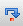# 序列与序表

## 序列是有序的泛型集合

 A 1 [] 2 [5,6,7] 3 [red,blue,yellow] 4 =["blue","yellow","white"] 5 =A3^A4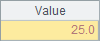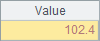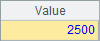A4中用表达式计算序列，和A3中的常数序列不同，表达式中的字符串需要用双引号扩出。A5计算A3A4中两个序列的交集，结果也是序列。A4A5中的结果如下：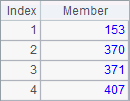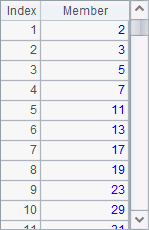A B 1 [] =[1,date("2014-05-01")] 2 [5,6,7] =["blue",[],[5,6,7]] 3 [red,blue,yellow] =["blue",A1,A2]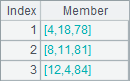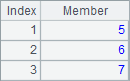A B 1 [Mike,Tom] [Tom,Mike] 2 =A1==B1

A2中可以用表达式=A1==B1判断两个序列是否相等，其结果为false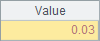A 1 [Mike,Tom] 2 =A1(2) 3 =A1.m(-1) 4 =A1.pos("Tom") 5 =A1.rvs()

A2中取出序列的第2个成员，也可以用=A1.m(2)来表示。A3中取出序列中倒数第1个成员。A4在序列中获得成员Tom的序号。A5将序列反转。A2,A3,A4A5中的结果如下：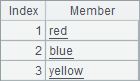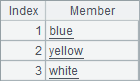A 1 =to(2,5)

A1中生成的序列如下：## 序表是有结构的序列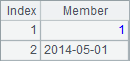A 1 =file("Order_Books.txt").import@t() 2 =demo.query("select * from CITIES") 3 =create(OrderID,Client,SellerId,Amount,OrderDate)

A1从文本文件生成序表，获得的结果就是上面的序表。A2通过SQL生成序表，A3指定字段名创建空序表。A2A3中的数据如下：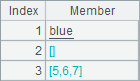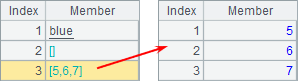A 1 =file("Order_Books.txt").import@t() 2 =A1.select(Amount>=20000 && month(Date)==5) 3 =A1.sort(SalesID,-Date) 4 =A1.groups(SalesID, month(Date); round(sum(Amount),2), count(~))

A2中查询出Amount字段大于等于20000Date5月的记录：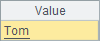A3将记录按照SalesID字段升序排列，SalesID一样时将按Date降序排列：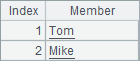A4SalesID和月份，对每一组数据的Amount求和，并计算该组订单计数：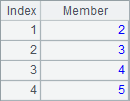A 1 =file("Order_Books.txt").import@t() 2 =A1.groups(month(Date); sum(decimal(string(Amount))):TotalAmount, count(~):Count) 3 =A2.derive(round(TotalAmount/TotalAmount[-1]-1,4): compValue)

A2中先统计出每月的总销售额：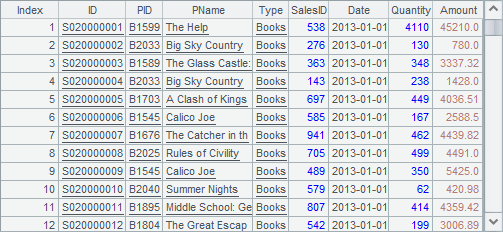A3中计算出最终结果如下：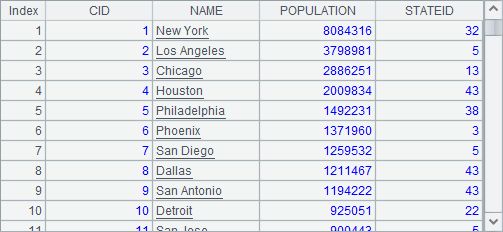A B 1 =file("Order_Books.txt").import@t() 2 Big =A1.select(Quantity>1000) 3 Importance =A1.select(Amount>10000) 4 =B2^B3 =A1\A4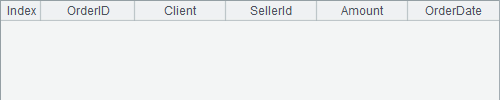B4中，用全部的订单与问题①的结果做差集，即可得到问题②的答案：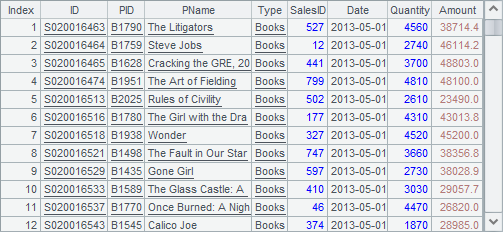## 排列是对序表记录的引用

 A B 1 =file("Order_Books.txt").import@t() =A1.to() 2 =A1.select(Amount>=20000 && month(Date)==5) =B1.select(Amount>=20000 && month(Date)==5) 3 =A1.sort(SalesID,-Date) =B1.sort(SalesID,-Date)

 A 1 =file("Order_Books.txt").import@t() 2 =A1.select(SalesID==363) 3 >A1.modify(3, 1:SalesID)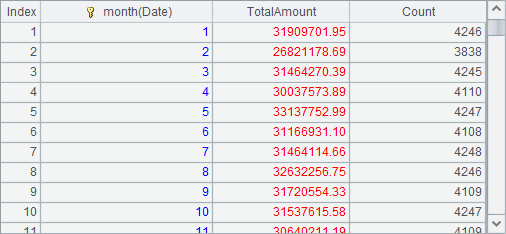A2从中选出所有SalesID363的记录，构成排列如下：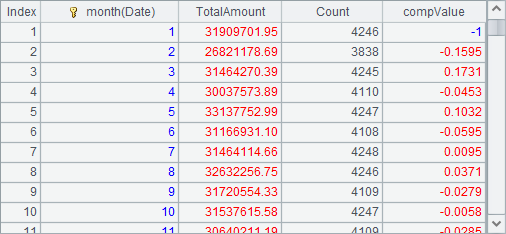A3中，修改了A1中的序表，修改了其中第3条记录，将其SalesID字段变为1。此时再次查看A1中的序表，可以发现第3条记录已经被修改：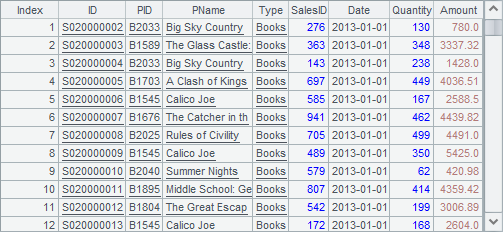-  TSeq.modify(5, 1000: Amount)  /OrderID=5的记录的Amount字段修改为1000，即小于2000

-  RSeq =TSeq.select(Amount>2000)  / OrderID=5的记录不会出现在RSeq中。

 A 1 =file("Order_Books.txt").import@t() 2 >A1.modify(3, 1:SalesID) 3 =A1.select(SalesID==363)